# Series-Parallel Combination AC Circuits

## AC Electric Circuits

• #### Question 1

 Don’t just sit there! Build something!!

Learning to mathematically analyze circuits requires much study and practice. Typically, students practice by working through lots of sample problems and checking their answers against those provided by the textbook or the instructor. While this is good, there is a much better way.

You will learn much more by actually building and analyzing real circuits, letting your test equipment provide the “answers” instead of a book or another person. For successful circuit-building exercises, follow these steps:

1. Carefully measure and record all component values prior to circuit construction.
2. Draw the schematic diagram for the circuit to be analyzed.
3. Carefully build this circuit on a breadboard or other convenient medium.
4. Check the accuracy of the circuit’s construction, following each wire to each connection point, and verifying these elements one-by-one on the diagram.
5. Mathematically analyze the circuit, solving for all voltage and current values.
6. Carefully measure all voltages and currents, to verify the accuracy of your analysis.
7. If there are any substantial errors (greater than a few percent), carefully check your circuit’s construction against the diagram, then carefully re-calculate the values and re-measure.

For AC circuits where inductive and capacitive reactances (impedances) are a significant element in the calculations, I recommend high quality (high-Q) inductors and capacitors, and powering your circuit with low frequency voltage (power-line frequency works well) to minimize parasitic effects. If you are on a restricted budget, I have found that inexpensive electronic musical keyboards serve well as “function generators” for producing a wide range of audio-frequency AC signals. Be sure to choose a keyboard “voice” that closely mimics a sine wave (the “panflute” voice is typically good), if sinusoidal waveforms are an important assumption in your calculations.

As usual, avoid very high and very low resistor values, to avoid measurement errors caused by meter “loading”. I recommend resistor values between 1 kΩ and 100 kΩ.

One way you can save time and reduce the possibility of error is to begin with a very simple circuit and incrementally add components to increase its complexity after each analysis, rather than building a whole new circuit for each practice problem. Another time-saving technique is to re-use the same components in a variety of different circuit configurations. This way, you won’t have to measure any component’s value more than once.

• #### Question 2

Calculate the line current and power factor in this AC power system:Now, calculate the line current and power factor for the same circuit after the addition of a capacitor in parallel with the load: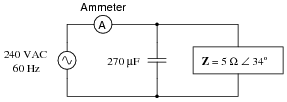• #### Question 3

It is often useful in AC circuit analysis to be able to convert a series combination of resistance and reactance into an equivalent parallel combination of conductance and susceptance, or visa-versa:We know that resistance (R), reactance (X), and impedance (Z), as scalar quantities, relate to one another trigonometrically in a series circuit. We also know that conductance (G), susceptance (B), and admittance (Y), as scalar quantities, relate to one another trigonometrically in a parallel circuit:If these two circuits are truly equivalent to one another, having the same total impedance, then their representative triangles should be geometrically similar (identical angles, same proportions of side lengths). With equal proportions, R/Z in the series circuit triangle should be the same ratio as G/Y in the parallel circuit triangle, that is R/Z = G/Y.

Building on this proportionality, prove the following equation to be true:

 Rseries Rparallel = Ztotal2

After this, derive a similar equation relating the series and parallel reactances (Xseries and Xparallel) with total impedance (Ztotal).

• #### Question 4

Determine an equivalent parallel RC network for the series RC network shown on the left:Note that I have already provided a value for the capacitor’s reactance (XC), which of course will be valid only for a particular frequency. Determine what values of resistance (R) and reactance (XC) in the parallel network will yield the exact same total impedance (ZT) at the same signal frequency.

• #### Question 5

Determine the total impedance of this series-parallel network by first converting it into an equivalent network that is either all-series or all-parallel: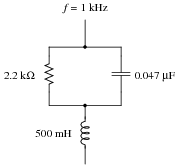• #### Question 6

Determine the equivalent parallel-connected resistor and inductor values for this series circuit:Also, express the total impedance of either circuit (since they are electrically equivalent to one another, they should have the same total impedance) in complex form. That is, express Z as a quantity with both a magnitude and an angle.

• #### Question 7

Determine the equivalent series-connected resistor and capacitor values for this parallel circuit:Also, express the total impedance of either circuit (since they are electrically equivalent to one another, they should have the same total impedance) in complex form. That is, express Z as a quantity with both a magnitude and an angle.

• #### Question 8

It is not uncommon to see impedances represented in AC circuits as boxes, rather than as combinations of R, L, and/or C. This is simply a convenient way to represent what may be complex sub-networks of components in a larger AC circuit:We know that any given impedance may be represented by a simple, two-component circuit: either a resistor and a reactive component connected in series, or a resistor and a reactive component connected in parallel. Assuming a circuit frequency of 250 Hz, determine what combination of series-connected components will be equivalent to this “box” impedance, and also what combination of parallel-connected components will be equivalent to this “box” impedance.

• #### Question 9

It is not uncommon to see impedances represented in AC circuits as boxes, rather than as combinations of R, L, and/or C. This is simply a convenient way to represent what may be complex sub-networks of components in a larger AC circuit:We know that any given impedance may be represented by a simple, two-component circuit: either a resistor and a reactive component connected in series, or a resistor and a reactive component connected in parallel. Assuming a circuit frequency of 700 Hz, determine what combination of series-connected components will be equivalent to this “box” impedance, and also what combination of parallel-connected components will be equivalent to this “box” impedance.

• #### Question 10

Calculate the amount of current through this impedance, and express your answer in both polar and rectangular forms:• #### Question 11

Determine the total impedance of this series-parallel network by first converting it into an equivalent network that is either all-series or all-parallel: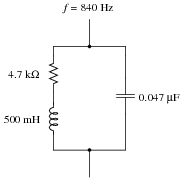• #### Question 12

Determine the voltage dropped between points A and B in this circuit:Hint: convert the parallel RC sub-network into a series equivalent first.

• #### Question 13

Determine the current through the series LR branch in this series-parallel circuit:Hint: convert the series LR sub-network into a parallel equivalent first.

• #### Question 14

Test leads for DC voltmeters are usually just two individual lengths of wire connecting the meter to a pair of probes. For highly sensitive instruments, a special type of two-conductor cable called coaxial cable is generally used instead of two individual wires. Coaxial cable - where a center conductor is “shielded” by an outer braid or foil that serves as the other conductor - has excellent immunity to induced “noise” from electric and magnetic fields: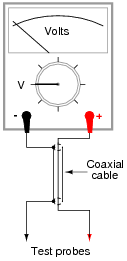When measuring high-frequency AC voltages, however, the parasitic capacitance and inductance of the coaxial cable may present problems. We may represent these distributed characteristics of the cable as “lumped” parameters: a single capacitor and a single inductor modeling the cable’s behavior: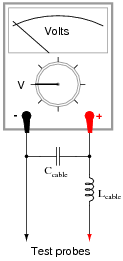Typical parasitic values for a 10-foot cable would be 260 pF of capacitance and 650 μH of inductance. The voltmeter itself, of course, is not without its own inherent impedances, either. For the sake of this example, let’s consider the meter’s “input impedance” to be a simple resistance of 1 MΩ.

Calculate what voltage the meter would register when measuring the output of a 20 volt AC source, at these frequencies:

f = 1 Hz ; Vmeter =
f = 1 kHz ; Vmeter =
f = 10 kHz ; Vmeter =
f = 100 kHz ; Vmeter =
f = 1 MHz ; Vmeter =
• #### Question 15

The voltage measurement range of a DC instrument may easily be “extended” by connecting an appropriately sized resistor in series with one of its test leads: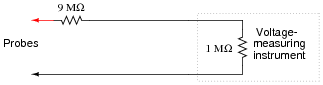In the example shown here, the multiplication ratio with the 9 MΩ resistor in place is 10:1, meaning that an indication of 3.5 volts at the instrument corresponds to an actual measured voltage of 35 volts between the probes.

While this technique works very well when measuring DC voltage, it does not do so well when measuring AC voltage, due to the parasitic capacitance of the cable connecting the test probes to the instrument (parasitic cable inductance has been omitted from this diagram for simplicity):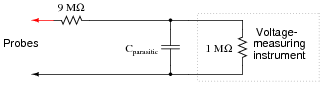To see the effects of this capacitance for yourself, calculate the voltage at the instrument input terminals assuming a parasitic capacitance of 180 pF and an AC voltage source of 10 volts, for the following frequencies:

f = 10 Hz ; Vinstrument =
f = 1 kHz ; Vinstrument =
f = 10 kHz ; Vinstrument =
f = 100 kHz ; Vinstrument =
f = 1 MHz ; Vinstrument =

The debilitating effect of cable capacitance may be compensated for with the addition of another capacitor, connected in parallel with the 9 MΩ range resistor. If we are trying to maintain a voltage division ratio of 10:1, this “compensating” capacitor must be 1/9 the value of the capacitance parallel to the instrument input:Re-calculate the voltage at the instrument input terminals with this compensating capacitor in place. You should notice quite a difference in instrument voltages across this frequency range!

f = 10 Hz ; Vinstrument =
f = 1 kHz ; Vinstrument =
f = 10 kHz ; Vinstrument =
f = 100 kHz ; Vinstrument =
f = 1 MHz ; Vinstrument =

Complete your answer by explaining why the compensation capacitor is able to “flatten” the response of the instrument over a wide frequency range.

• #### Question 16

Stereo (two-speaker) headphones typically use a plug with three contact points to connect the speakers to the audio amplifier. The three contact points are designated as “tip,” “ring,” and “sleeve” for reasons that are obvious upon inspection, and as such the plug is commonly referred to as a “TRS” plug. Both speakers in the headphone unit share a common connection (at the “sleeve” contact), with the “tip” and “ring” contacts providing connection to left and right speakers, respectively: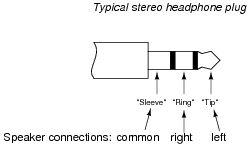Draw a picture showing how connections would be made to the plug’s contact points to form this circuit: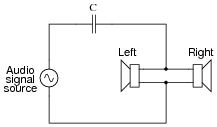• #### Question 17

Stereo (two-speaker) headphones typically use a plug with three contact points to connect the speakers to the audio amplifier. The three contact points are designated as “tip,” “ring,” and “sleeve” for reasons that are obvious upon inspection, and as such the plug is commonly referred to as a “TRS” plug. Both speakers in the headphone unit share a common connection (at the “sleeve” contact), with the “tip” and “ring” contacts providing connection to left and right speakers, respectively: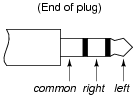Draw a picture showing how connections would be made to the plug’s contact points to form this circuit: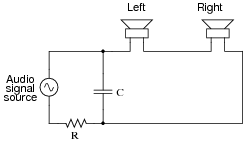• #### Question 18

Convert this series-parallel combination circuit into an equivalent simple-parallel circuit (all components connected in parallel with each other, with nothing in series), and also calculate the circuit’s total impedance: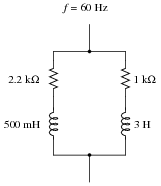• #### Question 19

Calculate the “output” voltage (Vout) for this AC circuit, expressed as a complex quantity in polar notation: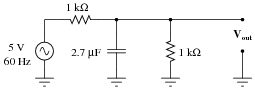• #### Question 20

It is not uncommon to see impedances represented in AC circuits as boxes, rather than as combinations of R, L, and/or C. This is simply a convenient way to represent what may be complex sub-networks of components in a larger AC circuit: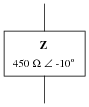We know that any given impedance may be represented by a simple, two-component circuit: either a resistor and a reactive component connected in series, or a resistor and a reactive component connected in parallel. Assuming a circuit frequency of 50 Hz, determine what combination of series-connected components will be equivalent to this “box” impedance, and also what combination of parallel-connected components will be equivalent to this “box” impedance.

• #### Question 21

It is not uncommon to see impedances represented in AC circuits as boxes, rather than as combinations of R, L, and/or C. This is simply a convenient way to represent what may be complex sub-networks of components in a larger AC circuit: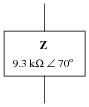We know that any given impedance may be represented by a simple, two-component circuit: either a resistor and a reactive component connected in series, or a resistor and a reactive component connected in parallel. Assuming a circuit frequency of 2 kHz, determine what combination of series-connected components will be equivalent to this “box” impedance, and also what combination of parallel-connected components will be equivalent to this “box” impedance.

• #### Question 22

Calculate the total impedance of this series network of impedances, in complex form:• #### Question 23

Calculate the total impedance of this parallel network of impedances, in complex form: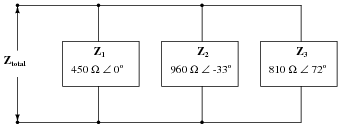• #### Question 24

Calculate the total impedance of this series-parallel network of impedances, in complex form: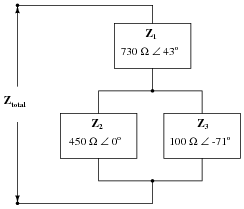• #### Question 25

Complete the table of values for this circuit, representing all quantities in complex-number form:• #### Question 26

Complete the table of values for this circuit, representing all quantities in complex-number form: